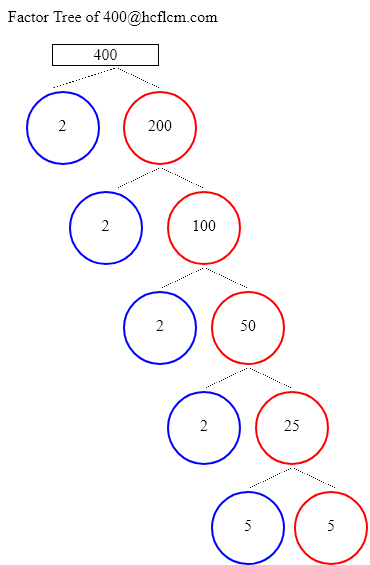# Factors of 400 | Find the Factors of 400 by Factoring Calculator

Factoring Calculator calculates the factors and factor pairs of positive integers.

Factors of 400 can be calculated quickly with the help of Factoring Calculator i.e. 1, 2, 4, 5, 8, 10, 16, 20, 25, 40, 50, 80, 100, 200, 400 positive integers that divide 400 without a remainder.

Factors of 400 are 1, 2, 4, 5, 8, 10, 16, 20, 25, 40, 50, 80, 100, 200, 400. There are 15 integers that are factors of 400. The biggest factor of 400 is 400.

Factors of:

### Factor Tree of 400 to Calculate the Factors

 400 2 200 2 100 2 50 2 25 5 5Factors of 400 are 1, 2, 4, 5, 8, 10, 16, 20, 25, 40, 50, 80, 100, 200, 400. There are 15 integers that are factors of 400. The biggest factor of 400 is 400.

Positive integers that divides 400 without a remainder are listed below.

• 1
• 2
• 4
• 5
• 8
• 10
• 16
• 20
• 25
• 40
• 50
• 80
• 100
• 200
• 400

### Factors of 400 in pairs

• 1 × 400 = 400
• 2 × 200 = 400
• 4 × 100 = 400
• 5 × 80 = 400
• 8 × 50 = 400
• 10 × 40 = 400
• 16 × 25 = 400
• 20 × 20 = 400
• 25 × 16 = 400
• 40 × 10 = 400
• 50 × 8 = 400
• 80 × 5 = 400
• 100 × 4 = 400
• 200 × 2 = 400
• 400 × 1 = 400

### Factors of 400 Table

FactorFactor Number
1one
2two
4four
5five
8eight
10ten
16sixteen
20twenty
25twenty five
40forty
50fifty
80eighty
100one hundred
200two hundred
400four hundred

### How to find Factors of 400?

As we know factors of 400 are all the numbers that can exactly divide the number 400 simply divide 400 by all the numbers up to 400 to see the ones that result in zero remainders. Numbers that divide without remainder are factors and in this case below are the factors

1, 2, 4, 5, 8, 10, 16, 20, 25, 40, 50, 80, 100, 200, 400 are the factors and all of them can exactly divide number 400.

### Frequently Asked Questions on Factors of 400

1. What are the factors of 400?

Answer: Factors of 400 are the numbers that leave a remainder zero. The ones that can divide 400 exactly i.e. factors are 1, 2, 4, 5, 8, 10, 16, 20, 25, 40, 50, 80, 100, 200, 400.

2.What are Factor Pairs of 400?

Answer:Factor Pairs of 400 are

• 1 × 400 = 400
• 2 × 200 = 400
• 4 × 100 = 400
• 5 × 80 = 400
• 8 × 50 = 400
• 10 × 40 = 400
• 16 × 25 = 400
• 20 × 20 = 400
• 25 × 16 = 400
• 40 × 10 = 400
• 50 × 8 = 400
• 80 × 5 = 400
• 100 × 4 = 400
• 200 × 2 = 400
• 400 × 1 = 400

3. What is meant by Factor Pairs?

Answer:Factor Pairs are numbers that when multiplied together will result in a given product.